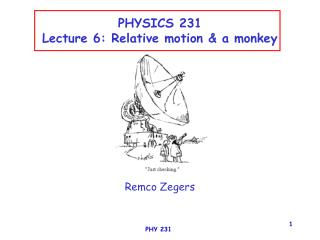Download PresentationPHYSICS 231 Lecture 6: Relative motion & a monkeyPHYSICS 231 Lecture 6: Relative motion & a monkey - PowerPoint PPT Presentation

Download PresentationPHYSICS 231 Lecture 6: Relative motion & a monkey
An Image/Link below is provided (as is) to download presentation

Download Policy: Content on the Website is provided to you AS IS for your information and personal use and may not be sold / licensed / shared on other websites without getting consent from its author. While downloading, if for some reason you are not able to download a presentation, the publisher may have deleted the file from their server.

- - - - - - - - - - - - - - - - - - - - - - - - - - - E N D - - - - - - - - - - - - - - - - - - - - - - - - - - -
Presentation Transcript

1. PHYSICS 231Lecture 6: Relative motion & a monkey Remco Zegers PHY 231

2. QUIZ (graded for extra credit) 3 quizpoints if correct 1 quizpoints if incorrect 5*(quizpoints collected during semester) extra credit= 0.7*3*(total number of quizzes) It’s okay to discuss with neighbor, but decide for yourself first. PHY 231

3. At the highest point the vertical component of the velocity is 0 but the horizontal velocity is still 1 m/s. Stop jumping already! • A fish jumps out of the water with a velocity of 4 m/s • in the vertical direction and 1 m/s in the horizontal direction. • What is his lowest speed while out of the water? • 0 m/s • 1 m/s • 3 m/s • 4 m/s • just give me 1 point PHY 231

4. Shoot the monkey The hunter aims his arrow exactly at the monkey At the moment he fires, the monkey drops off the branch. What happens? • hit • arrow goes over the monkey • arrow goes under the monley • no idea PHY 231

5. Always hit!! The hor. Position of the arrow is: x(t)=d-v0cos()t x(t)=0 at t=d/v0cos()=tx=0 Its vertical position is: y(t)=v0sin()t-0.5gt2 y(tx=0)=dtan()-0.5gt2=h-0.5gt2 h The vertical position of the monkey is: y(t)=h-0.5gt2 The horizontal position is 0  d PHY 231

6. Relative motion of 2 objects 20 km/h What is the velocity of the Ferrari relative to the tractor? And the other way around? 100 km/h A) 80 km/h & 80 km/h B) 20 km/h & -80 km/h C) 80 km/h & -80 km/h D) 100 km/h & 20 km/h PHY 231

7. d Recall of previous Lecture: if the plane is going at 100m/s, at what distance d from X should the plane drop the package? Relative motion of 2 objects II A UN plane drops a food package from a distance of 500 m high aiming at the dropzone X. What does the motion of the package look like from the point of view of a) the pilot b) the people at the drop zone 500m PHY 231

8. PHY 231

9. Horizontal direction: x(t)=x0+v0xt+0.5at2 d=100t Vertical direction: y(t)=y0+v0yt-0.5gt2 0=500-0.5gt2 t=10.1 s d=100*10.1=1010 m g Answer 100 m/s 500 m d? PHY 231

10. Cancel! Train (25 km/h) velocity of can parallel of the train (-25 km/h) velocity of the can perpendicular to the train (to the south) (10 km/h) Can out of the train A train is moving with a speed of 25 km/h to the east. An environment-unfriendly passenger throws a can out of the window. The velocity with which he throws the can relative to the moving train is 25 km/h toward the back of the train the (west) and 10 km/h away from the train toward the south. To an onlooker standing on the ground (south of the track), what is the observed direction of motion of the can? N W E S PHY 231

11. ( ) ( ) ( ) x3 y3 x2 y2 x1 y1 x3=4.7-5.4=-0.7 y3=6.6-3.2=3.4 = - vector subtraction Consider 2 vectors: r1=6.3 making an angle of 33o with the x-axis r2=8.1 making an angle of 55o with the x-axis What is the length and direction of r3=(r2-r1)? decompose: x1=r1cos1=6.3cos(33)=5.4 x2=r2cos2=8.1cos(55)=4.7 y1=r1sin 1=6.3sin(33)=3.2 y2=r2sin2=8.1sin(55)=6.6 Make sure to set your calculator to degrees! length of r3: ([-0.7]2+[3.4]2) = 3.5 angle with x-axis: 3=atan(y3/x3)=-78o!!!!!! add 180o: 102o (to get in right quadrant) PHY 231

12. T=282 M=230 W=91 plane in the wind I A plane, capable of flying at 230 mph with no wind, is moving at full speed in a gale blowing 91 mph from the east. The plane's ground speed is measured at 282 mph. What direction is the plane moving relative to the ground? (Give angle in degrees relative to an axis pointing east.) M=T-W (vectors!) Tx=282cos Ty=282sin Wx=91 Wy=0 Mx=Tx-Wx=282cos-91 My=Ty-Wy=282sin (Mx2+My2)=2302pythagorean theorem (282cos-91)2+(282sin)2=2302 2822cos2-2*91*282cos+912+2822sin2=2302 sin2+cos2=1 so cos=(2302-912-2822)/(-2*91*282) =47.2o so 180-47.2=132.8o relative to axis pointing east  PHY 231

13. b 282 c 230 91 a c2=a2+b2-2abcos  is the angle opposite to vector c. 2302=912+2822-2*91*282cos =47.20 so 180-47.2=132.80 relative to axis pointing east plane in the wind II A plane, capable of flying at 230 mph with no wind, is moving at full speed in a gale blowing 91 mph from the east. The plane's ground speed is measured at 282 mph. What direction is the plane moving relative to the ground? (Give angle in degrees relative to an axis pointing east.)  PHY 231